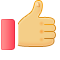# 附加3：跟着名著去探秘——英语六月June竟然是女神的名字？

《给孩子的希腊神话》是喵博士听听精心打造的又一力作，希望让孩子打开眼界，看到世界，在同龄人中脱颖而出。

## 用户评论(186)展示条数：20条20条50条100条

1396336frns

z z z z z z z z z z z z z z z z z z z z z z z z z z z z z z z z z z z z z z z z z z z z z z z z z z z z z z z z z z z z z z z z z z z z z z z z z z z z z z z z z z z z z z z z z z z z z z z z z z z z z z z z z z z z z z z z z z z z z z z z z z z z z z z z z z z z z z z z z z z z z z z z z z z z z z

13365925cyb